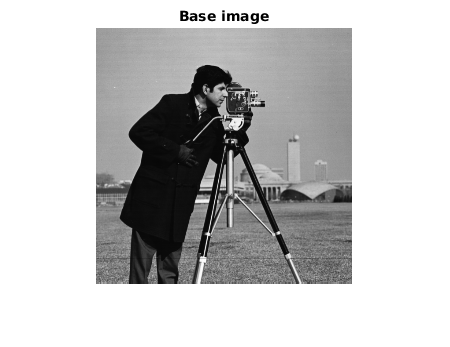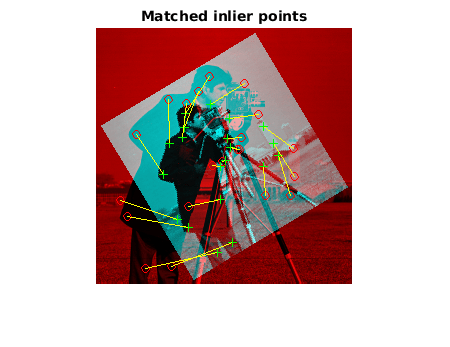# estimateGeometricTransform2D

Estimate 2-D geometric transformation from matching point pairs

## Syntax

``tform = estimateGeometricTransform2D(matchedPoints1,matchedPoints2,transformType)``
``````[tform,inlierIndex] = estimateGeometricTransform2D(___)``````
``[tform,inlierIndex,status] = estimateGeometricTransform2D(___)``
``[___] = estimateGeometricTransform2D(___, Name,Value)``

## Description

example

````tform = estimateGeometricTransform2D(matchedPoints1,matchedPoints2,transformType)` estimates a 2-D geometric transformation between two images by mapping the inliers in the matched points from one image `matchedPoints1` to the inliers in the matched points from another image `matchedPoints2`.```
``````[tform,inlierIndex] = estimateGeometricTransform2D(___)``` additionally returns a vector specifying each matched point pair as either an inlier or an outlier using the input arguments from the previous syntax.```
````[tform,inlierIndex,status] = estimateGeometricTransform2D(___)` additionally returns a status code indicating whether or not the function could estimate a transformation and, if not, why it failed. If you do not specify the `status` output, the function instead returns an error for conditions that cannot produce results.```
````[___] = estimateGeometricTransform2D(___, Name,Value)` specifies additional options using one or more name-value pair arguments in addition to any combination of arguments from previous syntaxes. For example, `'Confidence',99` sets the confidence value for finding the maximum number of inliers to `99`.```

## Examples

collapse all

Read an image and display it.

```original = imread('cameraman.tif'); imshow(original) title('Base Image')```Distort and display the transformed image.

```distorted = imresize(original,0.7); distorted = imrotate(distorted,31); figure imshow(distorted) title('Transformed Image')```Detect and extract features from the original and the transformed images.

```ptsOriginal = detectSURFFeatures(original); ptsDistorted = detectSURFFeatures(distorted); [featuresOriginal,validPtsOriginal] = extractFeatures(original,ptsOriginal); [featuresDistorted,validPtsDistorted] = extractFeatures(distorted,ptsDistorted);```

Match and display features between the images.

```index_pairs = matchFeatures(featuresOriginal,featuresDistorted); matchedPtsOriginal = validPtsOriginal(index_pairs(:,1)); matchedPtsDistorted = validPtsDistorted(index_pairs(:,2)); figure showMatchedFeatures(original,distorted,matchedPtsOriginal,matchedPtsDistorted) title('Matched SURF Points With Outliers');```Exclude the outliers, estimate the transformation matrix, and display the results.

```[tform,inlierIdx] = estimateGeometricTransform2D(matchedPtsDistorted,matchedPtsOriginal,'similarity'); inlierPtsDistorted = matchedPtsDistorted(inlierIdx,:); inlierPtsOriginal = matchedPtsOriginal(inlierIdx,:); figure showMatchedFeatures(original,distorted,inlierPtsOriginal,inlierPtsDistorted) title('Matched Inlier Points')```Use the estimated transformation to recover and display the original image from the distorted image.

```outputView = imref2d(size(original)); Ir = imwarp(distorted,tform,'OutputView',outputView); figure imshow(Ir); title('Recovered Image');```## Input Arguments

collapse all

Matched points from the first image, specified as either a `KAZEPoints` object, `cornerPoints` object, `SURFPoints` object, `MSERRegions` object, `ORBPoints` object, `BRISKPoints` object, or an M-by-2 matrix in which each row is a pair of [x,y] coordinates and M is the number of matched points.

Matched points from the second image, specified as either a `KAZEPoints` object, `cornerPoints` object, `SURFPoints` object, `MSERRegions` object, `ORBPoints` object, `BRISKPoints` object, or an M-by-2 matrix in which each row is a pair of [x,y] coordinates and M is the number of matched points.

Transformation type, specified as a character string. You can set the transform type to `'rigid'`, `'similarity'`, `'affine'`, or `'projective'`. Each transform type requires a minimum number of matched pairs of points to estimate a transformation. You can generally improve the accuracy of a transformation by using a larger number of matched pairs of points. This table shows the type of object associated with each transformation type and the minimum number of matched pairs of points the transformation requires.

Transformation Type`tform` ObjectMinimum Number of Matched Pairs of Points
`'rigid'``rigid2d`2
`'similarity'``affine2d`2
`'affine'``affine2d`3
`'projective'``projective2d`4

Data Types: `string`

### Name-Value Arguments

Specify optional pairs of arguments as `Name1=Value1,...,NameN=ValueN`, where `Name` is the argument name and `Value` is the corresponding value. Name-value arguments must appear after other arguments, but the order of the pairs does not matter.

Before R2021a, use commas to separate each name and value, and enclose `Name` in quotes.

Example: `'Confidence'`,`99` sets the confidence value for finding the maximum number of inliers to `99`.

Maximum number of random trials, specified as the comma-separated pair consisting of `'MaxNumTrials'` and a positive integer. This value specifies the number of randomized attempts the function makes to find matching point pairs. Specifying a higher value causes the function to perform additional computations, which increases the likelihood of finding inliers.

Data Types: `single` | `double` | `int8` | `int16` | `int32` | `int64` | `uint8` | `uint16` | `uint32` | `uint64`

Confidence of finding the maximum number of inliers, specified as the comma-separated pair consisting of `'Confidence'` and a positive numeric scalar in the range (0, 100). Increasing this value causes the function to perform additional computations, which increases the likelihood of finding a greater number of inliers.

Data Types: `single` | `double` | `int8` | `int16` | `int32` | `int64` | `uint8` | `uint16` | `uint32` | `uint64`

Maximum distance from a point to the projection of the corresponding point, specified as the comma-separated pair consisting of `'MaxDistance'` and a positive numeric scalar. `'MaxDistance'` specifies the maximum distance, in pixels, that a point can differ from the projected location of its corresponding point to be considered an inlier. The corresponding projection is based on the estimated transform.

The function checks for a transformation from `matchedPoints1` to `matchedPoints2`, and then calculates the distance between the matched points in each pair after applying the transformation. If the distance between the matched points in a pair is greater than the `'MaxDistance'` value, then the pair is considered an outlier for that transformation. If the distance is less than `'MaxDistance'`, then the pair is considered an inlier.Data Types: `single` | `double` | `int8` | `int16` | `int32` | `int64` | `uint8` | `uint16` | `uint32` | `uint64`

## Output Arguments

collapse all

Geometric transformation, returned as a `rigid2d`, `affine2d`, or `projective2d` object.

The returned geometric transformation matrix maps the inliers in `matchedPoints1` to the inliers in `matchedPoints2`. The function returns an object specific to the transformation type specified by the `transformType` input argument.

`transformType``tform`
`'rigid'``rigid2d`
`'similarity'``affine2d`
`'affine'``affine2d`
`'projective'``projective2d`

Status code, returned as `0`, `1`, or `2`. The status code indicates whether or not the function could estimate the transformation and, if not, why it failed.

ValueDescription
`0`No error
`1``matchedPoints1` and `matchedPoints2` inputs do not contain enough points
`2`Not enough inliers found

If you do not specify the `status` code output, the function returns an error if it cannot produce results.

Data Types: `int32`

Index of inliers, returned as an M-by-1 logical vector, where M is the number of point pairs. Each element contains either a logical `1` (`true`), indicating that the corresponding point pair is an inlier, or a logical `0` (`false`), indicating that the corresponding point pair is an outlier.

## Algorithms

The function excludes outliers using the M-estimator sample consensus (MSAC) algorithm. The MSAC algorithm is a variant of the random sample consensus (RANSAC) algorithm. Results may not be identical between runs due to the randomized nature of the MSAC algorithm.

 Hartley, Richard, and Andrew Zisserman. Multiple View Geometry in Computer Vision. 2nd ed. Cambridge, UK ; New York: Cambridge University Press, 2003.

 Torr, P.H.S., and A. Zisserman. “MLESAC: A New Robust Estimator with Application to Estimating Image Geometry.” Computer Vision and Image Understanding 78, no. 1 (April 2000): 138–56. https://doi.org/10.1006/cviu.1999.0832.